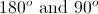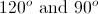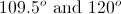# AP Chemistry : Molecular and Electronic Geometries

## Example Questions

← Previous 1 3

### Example Question #1 : Molecular And Electronic Geometries

What is the molecular shape of the following molecule?

SF6

seesaw

octahedral

hexahedral

trigonal bipyramidal

linear

octahedral

Explanation:

when a central atom of a molecule has 6 electron domains coming off of it (none of which are lone pairs of electrons), it is considered octahedral

### Example Question #1 : Molecular And Electronic Geometries

What is the shape of the SO2 molecule?

Linear

Trigonal planar

Octahedral

Bent

Square planar

Bent

Explanation:

The answer to this question can be determined by drawing a Lewis structure of the molecule. The S atom is central, with an O atom on each side. Adding in double bonds to each O atom and placing lone pairs on the O atoms give them each an octet, which leaves 2 more lone pairs of electrons to go on the S atom, creating a bent shape.

### Example Question #2 : Molecular And Electronic Geometries

VSEPR theory predicts that a BF3 molecule will be which of the following shapes?

Tetrahedral

Trigonal planar

Square planar

Bent

Trigonal planar

Explanation:

Molecules with three atoms around a central atom such as BF3 are trigonal planar because electron repulsion is minimized by positioning the three attachments toward the corners of an equilateral triangle.

### Example Question #1 : Molecular And Electronic Geometries

Which of the following is not a characteristic of a preferred Lewis structure?

Small or no formal charges

Less separation between opposite charges

Negative formal charges placed on more electronegative atoms

Multiple bonds between atoms

Multiple bonds between atoms

Explanation:

Multiple bonds, although they may be stronger, are not necessarily favored in a Lewis structure. It is dependent on the atoms involved. Thus, this is not a criterion used in determining a preferred Lewis structure.

### Example Question #1 : Molecular And Electronic Geometries

Consider the following reaction, which occurs at high temperatures with a silver catalyst: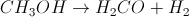What is the change in the geometry of the carbon atom between the reactant and the product?

Tetrahedral to trigonal pyramidal

Square planar to trigonal planar

Square planar to trigonal pyramidal

Trigonal planar to tetrahedral

Tetrahedral to trigonal planar

Tetrahedral to trigonal planar

Explanation:

In the reactant,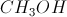, the carbon is bound to three hydrogens and one oxygen, and it has no lone pairs, so it has a tetrahedral geometry. In the product,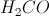, the carbon is bound to two hydrogens and one oxygen, so it must be double bonded to the oxygen in order to have a complete octet. Therefore, it has a trigonal planar geometry.

### Example Question #2 : Molecular And Electronic Geometries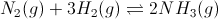Figure 1: Ammonia gas formation and equilibrium

What type of geometry does ammonia exhibit?

See-saw

Octahedral

Tetrahedral

Trigonal pyramidal

Bent

Trigonal pyramidal

Explanation:

Ammonia exhibits a tetrahedral electron pair geometry. It has three bonded pairs (between nitrogen and each hydrogen), and one lone pair (on nitrogen). This combination forms a trigonal pyramidal molecular geometry.

### Example Question #1 : Molecular And Electronic Geometries

For which of the following molecules are the molecular and electronic geometries NOT the same?

SO3

SiH4

PCl3

BeBr2

PCl3

Explanation:

The molecular geometry refers to the shape of the molecule's atoms, while the electronic geometry refers to the shape of the molecule's atoms and lone pair electrons. PCl3 has a trigonal pyramidal molecular geometry and a tetrahedral electronic geometry. All other answers have no lone pairs, and therefore have the same molecular and electronic geometries.

### Example Question #1 : Molecular And Electronic Geometries

What is the molecular geometry of ammonia (NH3)?

Trigonal planar

See-saw

Trigonal pyramidal

Tetrahedral

Trigonal pyramidal

Explanation:

This answer relies on remembering that ammonia has a lone electron pair in order to fill its valence shell. Without this lone pair, trigonal planar would be correct, however, since this electron pair is present, it repels the three hydrogens further away from a planar configuration. This results in a trigonal pyramidal shape.

### Example Question #1 : Molecular And Electronic Geometries

Which of the following compounds has an octahedral geometry?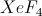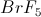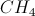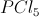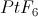Explanation:

The only answer choice that works is platinum hexafluoride. The platinum molecule is able to bind six fluorine molecules due to the availability of d orbitals in its valence shell. The octahedral shape looks like two pyramids with four sides each that have been stuck together by their bases. We can imagine the platinum at the middle with the six fluorines at each of the vertices of the pyramids. To have the octahedral shape, a molecule must have a central atom and six constituents.

### Example Question #4 : Molecular And Electronic Geometries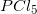has a trigonal bipyramidal geometry. What are the two bond angles present in?